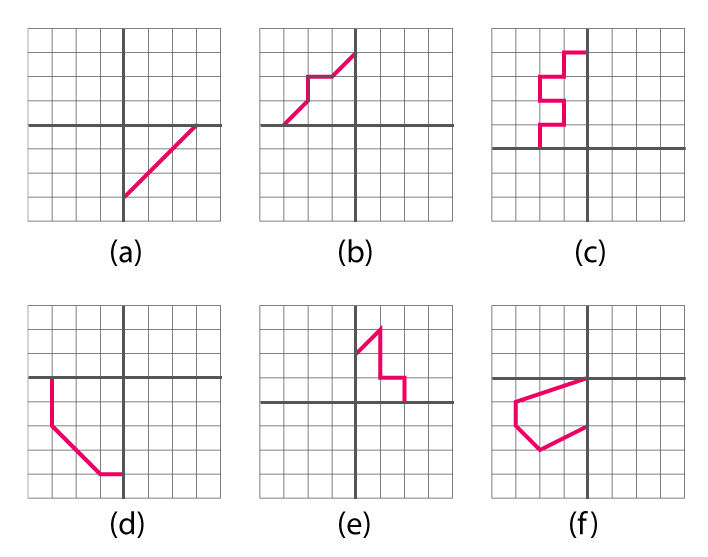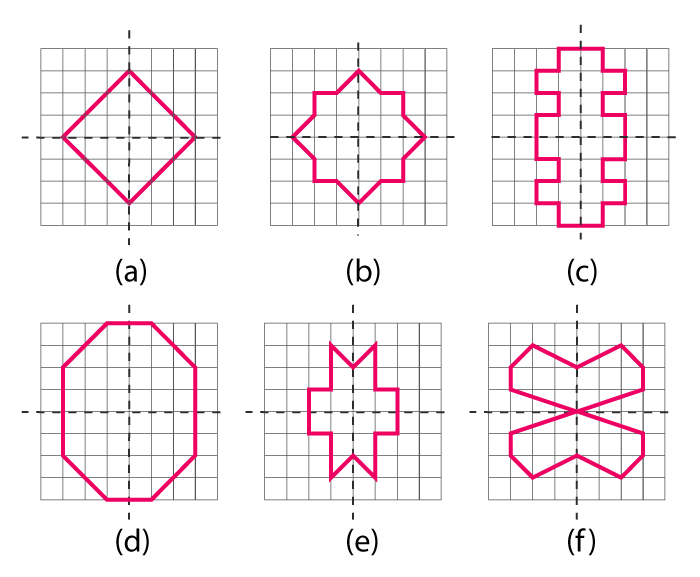# Copy the following drawing on squared paper. Complete each one of them such that the resulting figure has two dotted lines as two lines of symmetry. How did you go about completing the picture?Solutions:

We can complete the given figures by drawing similar parts as shown in these figures.

Let us first draw the vertical line of symmetry and then the horizontal line of symmetry. Or first about the horizontal line of symmetry and then about the vertical line of symmetry.

The figures shown below are the completed figures the given above(0)(0)